# Fractions + length - math problems

#### Number of problems found: 87

• Two ribbonsThe total length of the two ribbons is 13 meters. If one ribbon is 7 and 5/8 meters long, what is the length of the other ribbon?
• Conversion of units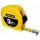Complete the following length data
• Length subtractingExpress in mm: 5 3/10 cm - 2/5 mm
• SeamstressThe seamstress cut the fabric into 3 parts. The first part was the eighth fabric, the second part was three-fifths of the fabric and the third part had a length of 66 cm. Calculate the original length of the fabric.
• Length 7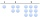Length equals 7/6 inch in width equals 7/9 inches the area is?
• Wiring 2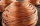Willie cut a piece of wire that was 3/8 of the total length of the wire. He cut another piece that was 9m long. The two pieces together were one half of the total length of the wire. How long was the wire before he cut it?
• Magic belt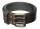The magic rectangular belt has the property that whenever its owner wants something, the length of the belt is reduced to 1/2 and the width to 1/3. After three such wishes, the belt had a content area 4 cm2. What was its original length if the original wi
• Iron pole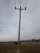The iron pole is in the ground 2/5 of its length, partly above the ground 1/3 is yellow, and the unpainted section is 6 m long. How long is the entire column?
• Wood 11Father has 12 1/5 meters long wood. Then I cut the wood into two pieces. One part is 7 3/5 meters long. Calculate the length of the other wood?
• Trapezoid thirdsThe ABCD trapezoid with the parallel sides of the AB and the CD and the E point of the AB side. The segment DE divides the trapezoid into two parts with the same area. Find the length of the AE line segment.
• MilimetersHow many millimeters is 1/4 meters?
• Semicircle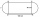Calculate the length of a semicircle with a radius of 6cm.
• Cube in a sphereThe cube is inscribed in a sphere with a volume 7253 cm3. Determine the length of the edges of a cube.
• Doug bikedDoug biked 5 1/4 miles in 3/4 of an hour. What is his average speed?
• Self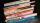A math text book is 2 2/9 inches thick. how many of these books will fit on a 120-inch self?
• PipeSteel pipe has a length 2.5 meters. About how many decimetres is 1/3 less than 4/8 of this steel pipe?
• Bar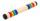Half of the bar is green, the third is yellow and the remaining 40cm is red. How long is a bar?
• GrandmaWe're going to Grandma. We still have 24 km left. How far does Grandma live if we have traveled from the total distance: half, a third, a fifth?
• Cyclist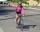Cyclist drives 5 km in half an hour, how long will drive in 3 hours?
• Walking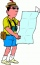Lucie can walk about 3 4/5 miles each hour. How far can she walk in 2 hours 45 minutes?

Do you have an interesting mathematical word problem that you can't solve it? Submit a math problem, and we can try to solve it.

We will send a solution to your e-mail address. Solved examples are also published here. Please enter the e-mail correctly and check whether you don't have a full mailbox.

Please do not submit problems from current active competitions such as Mathematical Olympiad, correspondence seminars etc...

Need help calculate sum, simplify or multiply fractions? Try our fraction calculator. Do you want to convert length units?